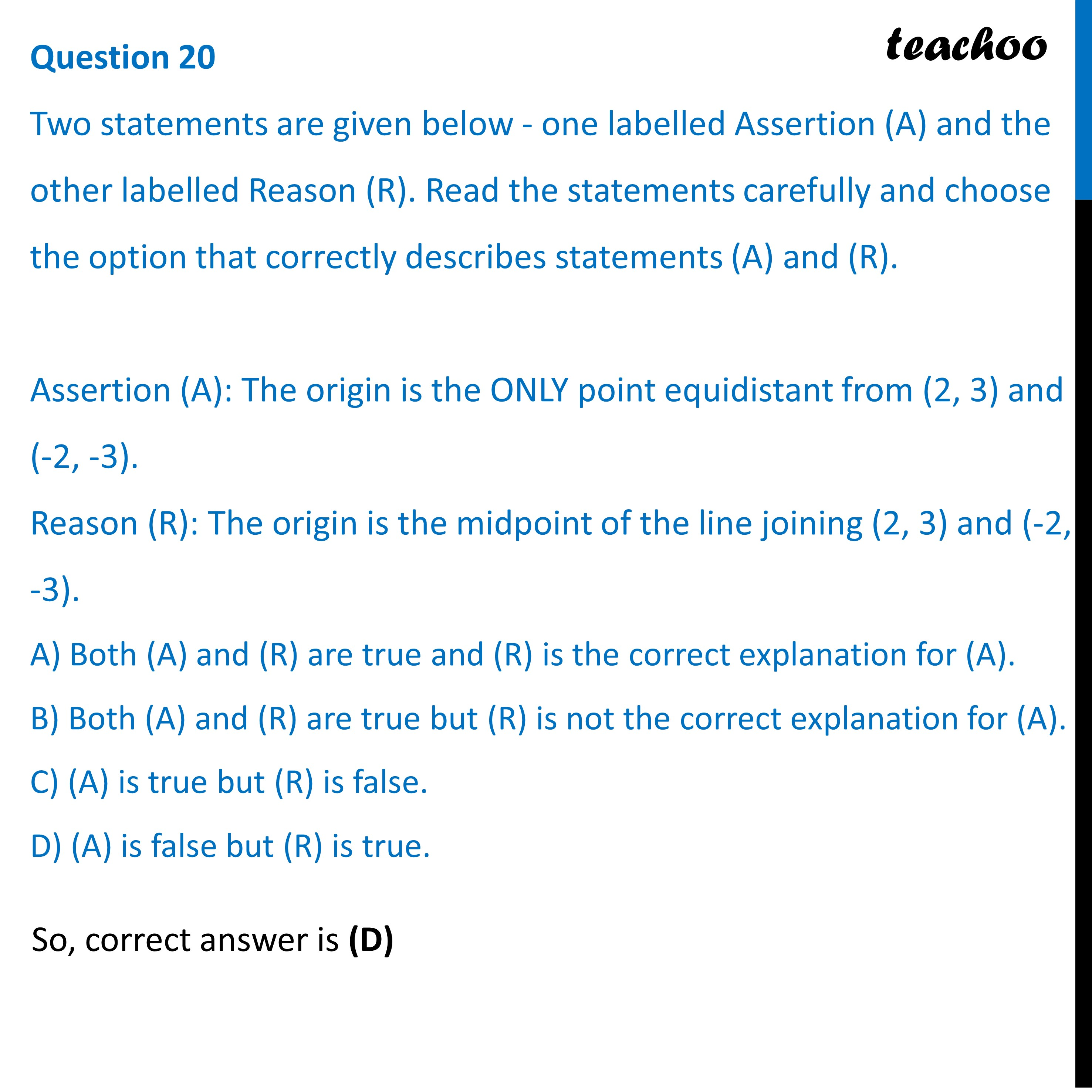Practice Questions CBSE - Maths Class 10 (2023 Boards)

Class 10
Solutions of Sample Papers for Class 10 Boards

## D) (A) is false but (R) is true.Learn in your speed, with individual attention - Teachoo Maths 1-on-1 Class

### Transcript

Two statements are given below - one labelled Assertion (A) and the other labelled Reason (R). Read the statements carefully and choose the option that correctly describes statements (A) and (R). Assertion (A): The origin is the ONLY point equidistant from (2, 3) and (-2, -3). Reason (R): The origin is the midpoint of the line joining (2, 3) and (-2, -3). A) Both (A) and (R) are true and (R) is the correct explanation for (A). B) Both (A) and (R) are true but (R) is not the correct explanation for (A). C) (A) is true but (R) is false. D) (A) is false but (R) is true.